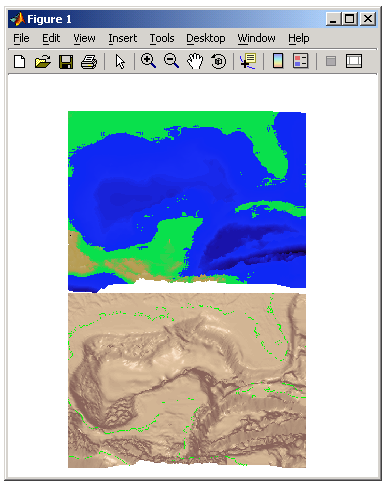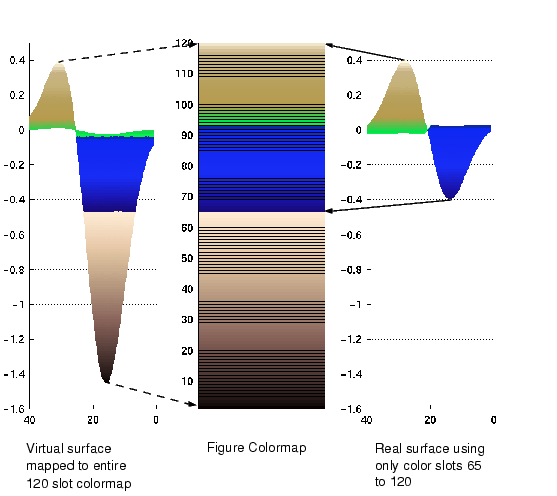GraphicsExample -- Simulating Multiple Colormaps in a Figure

Suppose you want to display two different surfaces in the same figure and color each surface with a different colormap. You can produce the effect of two different colormaps by concatenating two colormaps and then setting the `CLim` property of each axes to map into a different portion of the colormap.

This example creates two surfaces from the same topographic data. One uses the color scheme of a typical atlas -- shades of blue for the ocean and greens for the land. The other surface is illuminated with a light source to create the illusion of a three-dimensional picture. Such illumination requires a colormap that changes monotonically from dark to light.Calculating Color Limits

The key to this example is calculating values for `CLim` that cause each surface to use the section of the colormap containing the appropriate colors.

To calculate the new values for `CLim`, you need to know

• The total length of the colormap (`CmLength`)
• The beginning colormap slot to use for each axes (`BeginSlot)`
• The ending colormap slot to use for each axes (`EndSlot`)
• The minimum and maximum `CData` values of the graphic objects contained in the axes. That is, the values of the axes `CLim` property determined by MATLAB when `CLimMode` is `auto` (`CDmin` and `CDmax`).

First, define subplot regions and plot the surfaces.

• ```ax1 = subplot(2,1,1);
view([0 80])
surf(topodata)
shading interp
ax2 = subplot(2,1,2),;
view([0 80]);
surfl(topodata,[60 0])
shading interp
```

Concatenate two colormaps and install the new colormap.

• ```colormap([Lightingmap;Atlasmap]);
```

Obtain the data you need to calculate new values for `CLim`.

• ```CmLength   = size(get(gcf,'Colormap'),1);```% Colormap length
```BeginSlot1 = 1;          ```% Beginning slot
```EndSlot1   = size(Lightingmap,1); ```% Ending slot
```BeginSlot2 = EndSlot1+1;
EndSlot2   = CmLength;
CLim1      = get(ax1,'CLim');```% CLim values for each axis
```CLim2      = get(ax2,'CLim');
```

Defining a Function to Calculate CLim Values

Computing new values for `CLim` involves determining the portion of the colormap you want each axes to use relative to the total colormap size and scaling its `Clim` range accordingly. You can define a MATLAB function to do this.

• ```function CLim = newclim(BeginSlot,EndSlot,CDmin,CDmax,CmLength)
```%                Convert slot number and range
``````%                to percent of colormap
```PBeginSlot    = (BeginSlot - 1) / (CmLength - 1);
PEndSlot      = (EndSlot - 1) / (CmLength - 1);
PCmRange      = PEndSlot - PBeginSlot;
```%                Determine range and min and max
``````%                of new CLim values
```DataRange     = CDmax - CDmin;
ClimRange     = DataRange / PCmRange;
NewCmin       = CDmin - (PBeginSlot * ClimRange);
NewCmax       = CDmax + (1 - PEndSlot) * ClimRange;
CLim          = [NewCmin,NewCmax];
```

The input arguments are identified in the bulleted list above. The M-file first computes the percentage of the total colormap you want to use for a particular axes (`PCmRange`) and then computes the `CLim` range required to use that portion of the colormap given the `CData` range in the axes. Finally, it determines the minimum and maximum values required for the calculated `CLim` range and returns these values. These values are the color limits for the given axes.

Using the Function

Use the `newclim` M-file to set the `CLim` values of each axes. The statement

• ```set(ax1,'CLim',`new`clim(65,120,clim1(1),clim1(2)))
```

sets the `CLim` values for the first axes so the surface uses color slots 65 to 120. The lit surface uses the lower 64 slots. You need to reset its `CLim` values as well.

• ```set(ax2,'CLim',`new`clim(1,64,clim1(1),clim1(2)))
```

How the Function Works

MATLAB enables you to specify any values for the axes `CLim` property, even if these values do not correspond to the `CData` of the graphics objects displayed in the axes. MATLAB always maps the minimum `CLim` value to the first color in the colormap and the maximum `CLim` value to the last color in the colormap, whether or not there are really any `CData` values corresponding to these colors. Therefore, if you specify values for `CLim` that extend beyond the object's actual `CData` minimum and maximum, MATLAB colors the object with only a subset of the colormap.

The `newclim` M-file computes values for `CLim` that map the graphics object's actual `CData` values to the beginning and ending colormap slots you specify. It does this by defining a "virtual" graphics object having the computed `CLim` values. The following picture illustrates this concept. It shows a side view of two surfaces to make it easier to visualize the mapping of color to surface topography. The virtual surface is on the left and the actual surface on the right. In the center is the figure's colormap.

The real surface has `CLim` values of `[0.4 `-`0.4]`. To color this surface with slots 65 to 120, `newclim` computed new `CLim` values of `[0.4 `-`1.4269]`. The virtual surface on the left represents these values.Axes Color Limits -- the CLim Property Defining the Color of Lines for Plotting© 1994-2005 The MathWorks, Inc.